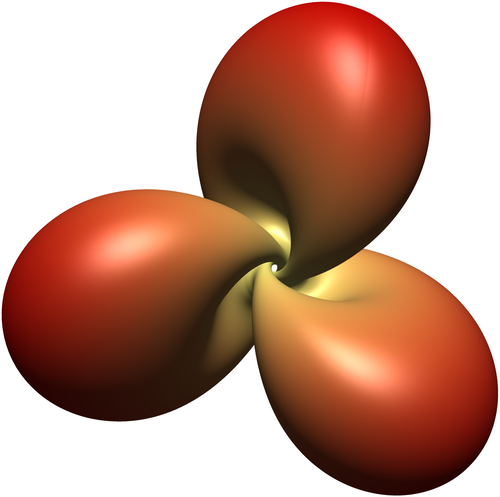# The Hopf torus of the tennis ball curve

Posted on August 30, 2018 by Stéphane Laurent

In a previous post I explain the construction of the Hopf torus of a closed curve on the sphere, and I show the rendering for a certain spherical curve.

On Paul Bourke’s site, I’ve found another spherical curve for which the corresponding Hopf torus is prettier.

This spherical curve is defined by the three coordinates $\begin{cases} p_1(t) & = & \sin\bigl(\pi/2 - (\pi/2 - A) \cos(t)\bigr) \cos\bigl(t/n + A \sin(2t)\bigr) \\ p_2(t) & = & \sin\bigl(\pi/2 - (\pi/2 - A) \cos(t)\bigr) \sin\bigl(t/n + A \sin(2t)\bigr) \\ p_3(t) & = & \cos\bigl(\pi/2 - (\pi/2 - A) \cos(t)\bigr) \end{cases}$ for $$t$$ varying from $$0$$ to $$2n\pi$$.

This is this curve:

For $$n=2$$ and $$A \approx 0.44$$ it looks like the curve appearing on a tennis ball.

For $$n=3$$ and $$A = 0.44$$, here is the correspondiing Hopf torus (rendered with Asymptote):The parameter $$n$$ defines the number of lobes of the torus. You can play with it below.